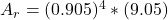## A damped oscillator is released from rest with an initial displacement of 10.00 cm. At the end of the first complete oscillation, the displa

Question

A damped oscillator is released from rest with an initial displacement of 10.00 cm. At the end of the first complete oscillation, the displacement reaches 9.05 cm. When 4 more oscillations are completed, what is the displacement reached

in progress 0
5 months 2021-08-09T15:16:50+00:00 1 Answers 20 views 0

The  displacement is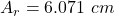Explanation:

From the question we are told that

The initial displacement is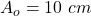The displacement at the end of first oscillation isGenerally the damping constant of this damped oscillator is mathematically represented as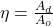substituting values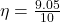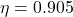The displacement after 4 more oscillation is mathematically represented as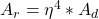substituting values